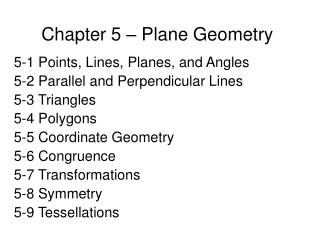DownloadDownload PresentationChapter 5 – Plane Geometry

# Chapter 5 – Plane Geometry

Download Presentation## Chapter 5 – Plane Geometry

- - - - - - - - - - - - - - - - - - - - - - - - - - - E N D - - - - - - - - - - - - - - - - - - - - - - - - - - -
##### Presentation Transcript

1. Chapter 5 – Plane Geometry 5-1 Points, Lines, Planes, and Angles 5-2 Parallel and Perpendicular Lines 5-3 Triangles 5-4 Polygons 5-5 Coordinate Geometry 5-6 Congruence 5-7 Transformations 5-8 Symmetry 5-9 Tessellations

2. 5-1 Points, Lines, Planes & Angles Vocabulary • Point – Names a location • Line – Perfectly straight and extends in both directions forever • Plane - Perfectly flat surface that extends forever in all directions • Segment – Part of a line between two points • Ray – Part of a line that starts at a point and extends forever in one direction

3. Point

4. Line

5. Segment

6. Ray

7. Example 1 • Name four points • Name the line • Name the plane • Name four segments • Name five rays

8. More Vocabulary • Right Angle – Measures exactly 90° • Acute Angle – Measures less than 90 ° • Obtuse Angle – Measures more than 90 ° • Complementary Angle – Angles that measure 90 ° together • Supplementary Angle – Angles that measure 180 ° together

9. Right Angle

10. Acute Angle

11. Obtuse Angle

12. Complementary Angle

13. Supplementary Angle

14. Example 2 • Name the following: • Right Angle • Acute Angle • Obtuse Angle • Complementary Angle • Supplementary Angle

15. Even MORE Vocabulary • Congruent – Figures that have the same size AND shape • Vertical Angles • Angles A & C are VA • Angles B & D are VA • If Angle A is 60° what is the measure of angle B?

16. Homework/Classwork Page 225, #13-34

17. 5-2 Parallel and Perpendicular Lines Vocabulary • Parallel Lines – Two lines in a plane that never meet, ex. Railroad Tracks • Perpendicular Lines – Lines that intersect to form Right Angles • Transversal – A line that intersects two or more lines at an angle other than a Right Angle

18. Parallel Lines

19. Perpendicular Lines

20. Transversal

21. Transversals to parallel lines have interesting properties • The color coded numbers are congruent

22. Properties of Transversals to Parallel Lines • If two parallel lines are intersected by a transversal: • The acute angles formed are all congruent • The obtuse angles are all congruent • And any acute angle is supplementary to any obtuse angle • If the transversal is perpendicular to the parallel lines, all of the angles formed are congruent 90° angles

23. Alternate Interior Angles

24. Alternate Exterior Angles

25. Corresponding Angles

26. Symbols • Parallel • Perpendicular • Congruent

27. Example 1 • In the figure Line X Y • Find each angle measure

28. In the figure Line A B • Find each angle measure

29. Homework/Classwork • Page 230, # 6-20

30. Triangle Sum Theorem – The angle measures of a triangle in a plane add to 180° Because of alternate interior angles, the following is true: 5-3 Triangles

31. Vocabulary • Acute Triangle – All angles are less than 90° • Right Triangle – Has one 90° angle • Obtuse Triangle – Has one obtuse angle

32. Example • Find the missing angle

33. Example • Find the missing angle.

34. Example • Find the missing angles

35. Vocabulary • Equilateral Triangle – 3 congruent sides and angles • Isosceles Triangle – 2 congruent sides and angles • Scalene Triangle – No congruent sides or angles

36. Equilateral Triangle • Isosceles Triangle • Scalene Triangle

37. Remember…they are ALL triangles

38. Example • Find the missing angle(s)

39. Example • Find the missing angle(s)

40. Example • Find the missing angle(s)

41. Example • Find the angles. Hint, remember the triangle sum theorem

42. Classwork/Homework • Page 237, #10-26

43. 5-4 Polygons • Polygons • Have 3 or more sides • Named by the number of sides • “Regular Polygon” means that all the sides are equal length

44. Finding the sum of angles in a polygon • Step 1: • Divide the polygon into triangles with common vertex

45. Step 2: • Multiply the number of triangles by 180

46. The Short Cut • 180°(n – 2) where n = the number of angles in the figure • In this case n = 6 • = 180°(6 – 2) • = 180°(4) • = 720° *Notice that n - 2 = 4 **Also notice that the figure can be broken into 4 triangles…coincidence? I don’t think so!